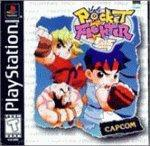# Pocket Fighter (e)

Andere Lösungen
```======================
Pocket Fighter for PSX
======================

Move and Flash combo list
Version 1.1 by Ian Ronan A. Viray-KIT
e-mail: speed@philonline.net
June 19, 1998

Contents

1. Hidden Characters?
2. Character Movelist
3. Flash Combo
___________________________________________________________________________
___

1. Hidden Characters?

Gouki (Akuma) and Dan are not seen on the character select screen, but
they are there. All you have to do is move the cursor over Ryu and then
move left and you've found Gouki. To the right of Ken is Dan. That
wasn't that hard now was it?

2. Movelist

The following moves are those that I have found thus far. For some of
them I
don't have the official name, but I do have a shot description of what
they
looks like.

Key:

FCF= Full Circle Forward                         P= Punch
HCF= Half Circle Foward                          K= Kick
HCB= Half Circle Backward                        S= Special
QCF= Quarter Circle Foward                       T= Taunt
QCB= Quarter Circle Backward
(F)DP= Foward Dragon Punch (f,d,df)
(B)DP= Backward Dragon Punch (b,d,db)

Chun Li
-------
QCF+P= Kikoken (fireball)
(F)DP+K= Rising Hurricane (upward spin kick)
Rapid K= Lightning Kick
HCF+K= Hurricane Kick (forward spin kick)
QCF+S= Super Kikoken
HCB+S= Bicycle Attack
(F)DP+S= Super Rising Hurricane
P+K= Throw
HCF+P+K= Love Letter Throw

Dan
---
(F)DP+P= Shoryuken (rising uppercut)
QCB+K= Flying Kick
HCF+S= Proton Cannon
(F)DP+S= Super Shoryuken
P+K= Throw
HCF+P+K= Oponent turns to stone (follow up with another hit)
HCB+P+K= Launch Throw
Down+T= Silly Taunt

Felicia
-------
QCF+P= Catroll charge
QCB+P= Double Catball Hop
(While in D.C.H.) Rapid P= Cat scratch (5 hit max)
QCF+K= Side Swipe
(F)DP+K= Delta Kick
HCF+S= Help! (multiple cat attack)
HCB+S= Feline Kickline
(F)DP+S= Lightning Ball Attack
P+K= 9 Hit Scratch
FCF+P+K= Zangief Style Smash
HCF+P+K= Foward Throw
HCB+P+K= 5 Hit Lick

Gouki(Akuma)
------------
(F)DP+P= Shoryuken (rising uppercut)
QCB+K= Hurricane Kick (spin kick)
(F)DP+K= Teleport Foward
(B)DP+K= Teleport Backward
HCB+S= Teleport Attack
(F)DP+S= Super Shoryuken
P+K= One Hand Throw
(B)DP+P+K= Low Launch Throw
HCB+P+K= High Launch Throw
HCF+P+K= Turn Opponent to Stone (use a follow-up hit)

Ibuki
-----
QCF+P= Cammy Knife Throw
(F)DP+K= Rising Kick
(B)DP+K= Penguin Attack
QCB+K= Spin Kick
QCF+S= Super Cammy
HCB+S= Frog Flame Attack
(F)DP+S= Slice and Dice
P+K= Disappearing Act/ Air Toss
HCB+P+K= Top Spin
HCF+P+K= High Air Toss

Ken
---
(F)DP+P= Shoryuken (rising uppercut)
QCB+K= Hurricane Kick (spin kick)
(F)DP+S= Super Shoryuken
(B)DP+S= Pheonix Shoryuken
HCF+S= Super Hurricane Kick
P+K= One Hand Throw
HCF+P+K= Football/Soccer Kick
HCB+P+K= High Launch Throw

Lei-Lei (Hsien-Ko)
------------------
QCF+P= Weapon Throw
QCB+P= Reflect Gong
HCF+K= Rising Swords
HCB+S= Spike Ball Drop
QCF+S= Circus Ball Ride
(F)DP+S= Umbrella Attack
P+K= Throw Behind
HCF+P+K= Air Toss/Opponent Lands on Spinning Blades
HCB+P+K= High Throw Foward
(While in Air) FF or BB= Walk on Air

Morrigan
--------
(F)DP+P= Wing Uppercut
QCB+K= Spin Attack
HCF+S= Lillith & Morrigan Combo
(F)DP+S= Super Shoryuken
QCB+S= Super Spin Attack
P+K= Ground Smash
HCF+P+K= Jetpack Ground Smash
HCB+P+K= Cannon Launch

Ryu
---
(F)DP+P= Shoryuken (Rising Uppercut)
QCB+K= Hurricane Kick (Spin Kick)
QCB+S= Super Hurricane Kick
(F)DP+S= Super Shoryuken
P+K= One Hand Throw
(B)DP+P+K= Low Launch Throw
HCB+P+K= High Launch Throw
HCF+P+K= 11 Hit Pound

Sakura
------
(F)DP+P= Umbrella Shoryuken
HCF+K= Baby Attack
(F)DP+S= Super Umbrella Shoryuken
HCB+S= Hunk Attack
HCF+P+K= Bunny Butt Bump
HCB+P+K= High Toss Foward

Tabasa(Tessa)
-------------
QCF+P= Dragon Strike
(F)DP+P= Levitation Attack
QCF+K= Dragon Roll
QCF+S= Proton Cannon
HCB+S= Robot Attack
(F)DP+S= Dragon Transformation
P+K= Cat Attack
HCF+P+K= Super Cat Attack
HCB+P+K= Rocket Launch

Zangief
-------
(F)DP+P= Flaming Palm Strike
P+S= Spin Attack
(F)DP+S= Russian Dance
HCF+S= Foward Jump and Bite
FCF+S= Double Suplex and Smash
P+K= Bite
FCF+P+K= Spinning Smash
HCF+P+K= Suplex
HCB+P+K= High Throw

3. Flash Combos

The flash combo is simply a series of hits on an opponent. They must
always be
started with a punch and then can be followed-up by a series of punches
or
kicks. When you execute a flash combo, your character will  (usually)
change
costume with each blow. In the combo of (p,p,p,k), (p,p,k,k), (p,k,p,p),
and
(p,k,k,p) the last hit is always unblockable.  I have made a combo
description
list  for each character to help.

The combo tree looks like this:

punch----punch
/     \  /
/       \/
punch        /\
/     \      /  \
/       \    /    \
/         kick------kick    (unblockable)
punch
\        punch----punch     (unblockable)
\      /     \  /
\    /       \/
kick        /\
\      /  \
\    /    \
kick------kick
```